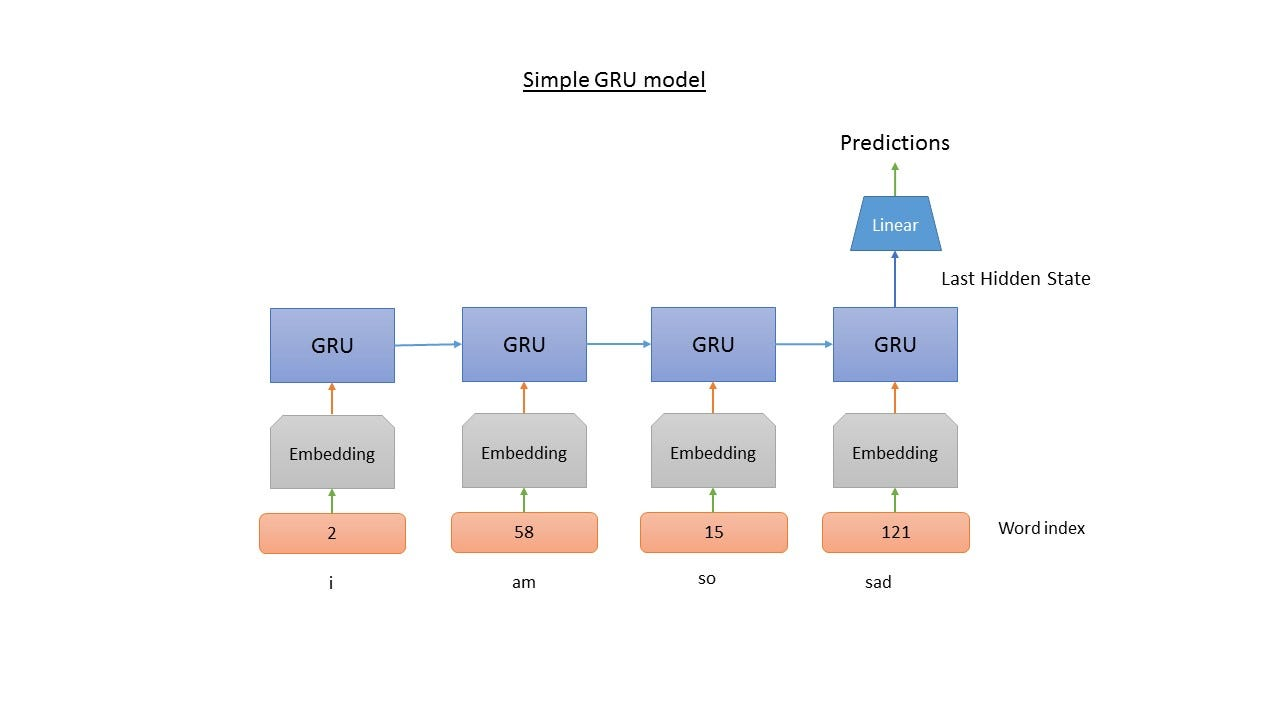## Assignment 2.3: Text classification via RNN (30 points)¶

In this assignment you will perform sentiment analysis of the IMDBs reviews by using RNN. An additional goal is to learn high abstactions of the torchtext module that consists of data processing utilities and popular datasets for natural language.

In [ ]:
import pandas as pd
import numpy as np
import torch

from torchtext import datasets

from torchtext.data import Field, LabelField
from torchtext.data import BucketIterator

import torch.nn as nn
import torch.nn.functional as F
import torch.optim as optim


### Preparing Data¶

In [ ]:
TEXT = Field(sequential=True, lower=True)
LABEL = LabelField()

In [ ]:
train, tst = datasets.IMDB.splits(TEXT, LABEL)
trn, vld = train.split()

In [ ]:
%%time
TEXT.build_vocab(trn)

In [ ]:
LABEL.build_vocab(trn)


The vocab.freqs is a collections.Counter object, so we can take a look at the most frequent words.

In [ ]:
TEXT.vocab.freqs.most_common(10)


### Creating the Iterator (2 points)¶

During training, we'll be using a special kind of Iterator, called the BucketIterator. When we pass data into a neural network, we want the data to be padded to be the same length so that we can process them in batch:

e.g. [ [3, 15, 2, 7], [4, 1], [5, 5, 6, 8, 1] ] -> [ [3, 15, 2, 7, 0], [4, 1, 0, 0, 0], [5, 5, 6, 8, 1] ]

If the sequences differ greatly in length, the padding will consume a lot of wasteful memory and time. The BucketIterator groups sequences of similar lengths together for each batch to minimize padding.

Complete the definition of the BucketIterator object

In [ ]:
train_iter, val_iter, test_iter = BucketIterator.splits(
(trn, vld, tst),
batch_sizes=(64, 64, 64),
sort=False,
sort_within_batch=False,
device='cuda',
repeat=False
)


Let's take a look at what the output of the BucketIterator looks like. Do not be suprised batch_first=True

In [ ]:
batch = next(train_iter.__iter__()); batch.text


The batch has all the fields we passed to the Dataset as attributes. The batch data can be accessed through the attribute with the same name.

In [ ]:
batch.__dict__.keys()


### Define the RNN-based text classification model (10 points)¶

Start simple first. Implement the model according to the shema below.In [ ]:
class RNNBaseline(nn.Module):
def __init__(self, hidden_dim, emb_dim):
super().__init__()
# =============================
#      Write code here
# =============================

def forward(self, seq):
# =============================
#      Write code here
# =============================
return preds

In [ ]:
em_sz = 200
nh = 300
model = RNNBaseline(nh, emb_dim=em_sz); model


If you're using a GPU, remember to call model.cuda() to move your model to the GPU.

In [ ]:
model.cuda()


### The training loop (3 points)¶

Define the optimization and the loss functions.

In [ ]:
opt = # your code goes here
loss_func = # your code goes here


Define the stopping criteria.

In [ ]:
epochs = # your code goes here

In [ ]:
%%time
for epoch in range(1, epochs + 1):
running_loss = 0.0
running_corrects = 0
model.train()
for batch in train_iter:

x = batch.text
y = batch.label

preds = model(x)
loss = loss_func(preds, y)
loss.backward()
opt.step()
running_loss += loss.item()

epoch_loss = running_loss / len(trn)

val_loss = 0.0
model.eval()
for batch in val_iter:

x = batch.text
y = batch.label

preds = model(x)
loss = loss_func(preds, y)
val_loss += loss.item()

val_loss /= len(vld)
print('Epoch: {}, Training Loss: {}, Validation Loss: {}'.format(epoch, epoch_loss, val_loss))


### Calculate performance of the trained model (5 points)¶

In [ ]:
for batch in test_iter:
x = batch.text
y = batch.label


Write down the calculated performance

### Experiments (10 points)¶

Experiment with the model and achieve better results. You can find advices here. Implement and describe your experiments in details, mention what was helpful.